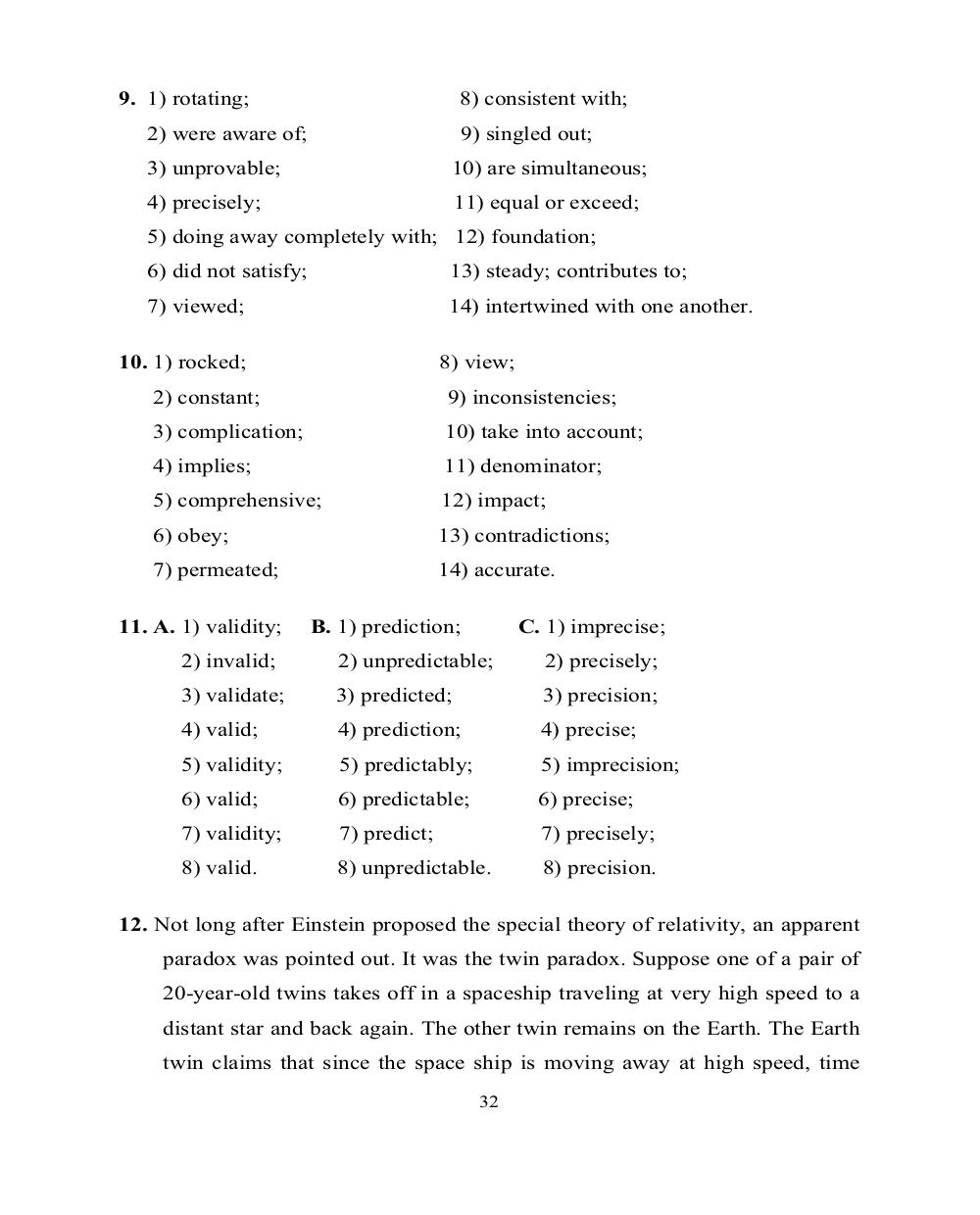# Английский язык. Ч.3 (Tests 10,11,12). Ильичева Н.А - 32 стр.

Составители:

Рубрика:

• ## Иностранный язык

9. 1) rotating; 8) consistent with;
2) were aware of; 9) singled out;
3) unprovable; 10) are simultaneous;
4) precisely; 11) equal or exceed;
5) doing away completely with; 12) foundation;
6) did not satisfy; 13) steady; contributes to;
7) viewed; 14) intertwined with one another.
10. 1) rocked; 8) view;
2) constant; 9) inconsistencies;
3) complication; 10) take into account;
4) implies; 11) denominator;
5) comprehensive; 12) impact;
7) permeated; 14) accurate.
11. A. 1) validity; B. 1) prediction; C. 1) imprecise;
2) invalid; 2) unpredictable; 2) precisely;
3) validate; 3) predicted; 3) precision;
4) valid; 4) prediction; 4) precise;
5) validity; 5) predictably; 5) imprecision;
6) valid; 6) predictable; 6) precise;
7) validity; 7) predict; 7) precisely;
8) valid. 8) unpredictable. 8) precision.
12. Not long after Einstein proposed the special theory of relativity, an apparent
paradox was pointed out. It was the twin paradox. Suppose one of a pair of
20-year-old twins takes off in a spaceship traveling at very high speed to a
distant star and back again. The other twin remains on the Earth. The Earth
twin claims that since the space ship is moving away at high speed, time
329. 1) rotating;                             8) consistent with;
2) were aware of;                         9) singled out;
3) unprovable;                          10) are simultaneous;
4) precisely;                           11) equal or exceed;
5) doing away completely with; 12) foundation;
6) did not satisfy;                     13) steady; contributes to;
7) viewed;                              14) intertwined with one another.

10. 1) rocked;                            8) view;
2) constant;                            9) inconsistencies;
3) complication;                       10) take into account;
4) implies;                            11) denominator;
5) comprehensive;                      12) impact;
7) permeated;                          14) accurate.

11. A. 1) validity;      B. 1) prediction;           C. 1) imprecise;
2) invalid;          2) unpredictable;          2) precisely;
3) validate;        3) predicted;               3) precision;
4) valid;            4) prediction;             4) precise;
5) validity;         5) predictably;            5) imprecision;
6) valid;            6) predictable;            6) precise;
7) validity;         7) predict;                7) precisely;
8) valid.           8) unpredictable.           8) precision.

12. Not long after Einstein proposed the special theory of relativity, an apparent
paradox was pointed out. It was the twin paradox. Suppose one of a pair of
20-year-old twins takes off in a spaceship traveling at very high speed to a
distant star and back again. The other twin remains on the Earth. The Earth
twin claims that since the space ship is moving away at high speed, time
32